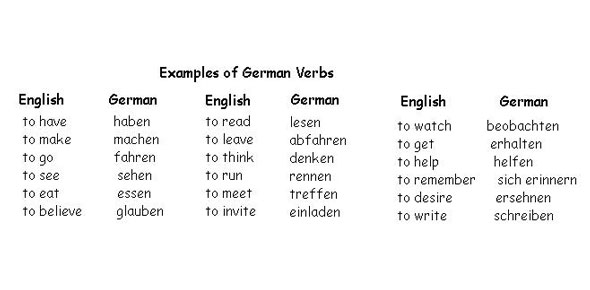# Unitechtransfer-german Centre For Automation And Robotics,Germany.

25 Questions | Attempts: 551
ShareSettings• 1.
Which is the correct operator for power(x^y)?
• A.

X^y

• B.

X**y

• C.

X^^y

• D.

None of the mentioned

• 2.
What is the output of the following? sentence = 'horses are fast' regex = re.compile('(?P\w+) (?P\w+) (?P\w+)') matched = re.search(regex, sentence) print(matched.groups())
• A.

{‘animal’: ‘horses’, ‘verb’: ‘are’, ‘adjective’: ‘fast’}

• B.

(‘horses’, ‘are’, ‘fast’)

• C.

‘horses are fast’

• D.

‘are’

• 3.
Which of the following cannot be a variable?
• A.

__init__

• B.

it

• C.

In

• D.

On

• 4.
What is the output of the following? x = "abcdef" i = "a" while i in x[1:]: print(i, end = " ")
• A.

A a a a a a

• B.

A

• C.

No output

• D.

Error

• 5.
What is the output of the following? for i in '': print (i)
• A.

None

• B.

(nothing is printed)

• C.

Error

• D.

None of the mentioned

• 6.
What is the output of the following code ?
1. >>>example = "helle"
2. >>>example.rfind("e")
• A.

4

• B.

3

• C.

1

• D.

-1

• 7.
What is the output when following code is executed ?
1. >>>print("D", end = ' ')
2. >>>print("C", end = ' ')
3. >>>print("B", end = ' ')
4. >>>print("A", end = ' ')
• A.

DCBA

• B.

A, B, C, D

• C.

D C B A

• D.

A, B, C, D will be displayed on four lines

• 8.
What is the output of the following?print("abcdef".center(10, '12'))
• A.

12abcdef12

• B.

Abcdef1212

• C.

1212abcdef

• D.

Error

• 9.
What is the output of the following?print("abcdef".find("cd") == "cd" in "abcdef")
• A.

True

• B.

False

• C.

Error

• D.

None of the mentioned.

• 10.
What is the output of the following?print('my_string'.isidentifier())
• A.

True

• B.

False

• C.

None

• D.

Error

• 11.
What is the output of the following?print('1.1'.isnumeric())
• A.

True

• B.

False

• C.

None

• D.

Error

• 12.
To shuffle the list(say list1) what function do we use ?
• A.

list1.shuffle()

• B.

Shuffle(list1)

• C.

Random.shuffle(list1)

• D.

Random.shuffleList(list1)

• 13.
What is the output when following code is executed ?
1. >>>"Welcome to Python".split()
• A.

[“Welcome”, “to”, “Python”]

• B.

(“Welcome”, “to”, “Python”)

• C.

{“Welcome”, “to”, “Python”}

• D.

“Welcome”, “to”, “Python”

• 14.
What will be the output?
1. values = [[3, 4, 5, 1], [33, 6, 1, 2]]
2.
3. v = values
4. for row in range(0, len(values)):
5. for column in range(0, len(values[row])):
6. if v < values[row][column]:
7. v = values[row][column]
8.
9. print(v)
• A.

3

• B.

5

• C.

6

• D.

33

• 15.
What will be the output?
1. d1 = {"john":40, "peter":45}
2. d2 = {"john":466, "peter":45}
3. d1 > d2
• A.

True

• B.

False

• C.

Error

• D.

None

• 16.
What will be the output?
1. >>>t=(1,2,4,3)
2. >>>t[1:-1]
• A.

(1, 2)

• B.

(1, 2, 4)

• C.

(2, 4)

• D.

(2, 4, 3)

• 17.
To open a file c:\scores.txt for writing, we use
• A.

Outfile = open(“c:\scores.txt”, “w”)

• B.

Outfile = open(“c:\\scores.txt”, “w”)

• C.

Outfile = open(file = “c:\scores.txt”, “w”)

• D.

Outfile = open(file = “c:\\scores.txt”, “w”)

• 18.
What is the output of this program?
1. fo = open("foo.txt", "rw+")
2. print "Name of the file: ", fo.name
3.
4. # Assuming file has following 5 lines
5. # This is 1st line
6. # This is 2nd line
7. # This is 3rd line
8. # This is 4th line
9. # This is 5th line
10.
11. for index in range(5):
12. line = fo.next()
13. print "Line No %d - %s" % (index, line)
14.
15. # Close opend file
16. fo.close()
• A.

Compilation Error

• B.

Syntax Error

• C.

Displays Output

• D.

None of the mentioned

• 19.
What is the output of this program?
1. import sys
2.
3. sys.stdout.write(' Hello\n')
4. sys.stdout.write('Python\n')
• A.

Compilation Error

• B.

Runtime Error

• C.

Hello Python

• D.

Hello Python

• 20.
What is the use of “w” in file handling?
• A.

• B.

Write

• C.

Append

• D.

None of the above

• 21.
What is the output of the below program?
1. def printMax(a, b):
2. if a > b:
3. print(a, 'is maximum')
4. elseif a == b:
5. print(a, 'is equal to', b)
6. else:
7. print(b, 'is maximum')
8. printMax(3, 4)
• A.

3

• B.

4

• C.

4 is maximum

• D.

None of the mentioned.

• 22.
What is called when a function is defined inside a class?
• A.

Module

• B.

Class

• C.

Another Function

• D.

Method

• 23.
What is the output of below program?
1. def f(x, y, z): return x + y + z
2.
3. f(2, 30, 400)
• A.

432

• B.

24000

• C.

430

• D.

None of the mentioned

• 24.
Which module in Python supports regular expressions?
• A.

Re

• B.

Regex

• C.

Pyregex

• D.

None of the mentioned

• 25.
Which of the following creates a pattern object?
• A.

re.create(str)

• B.

re.regex(str)

• C.

Re.compile(str)

• D.

Re.assemble(str)

## Related TopicsBack to top
×

Wait!
Here's an interesting quiz for you.